## 15.1.1 Stability

The subject of stability addresses properties of a vector field with respect to a given point. Letdenote a smooth manifold on which the vector field is defined;may be a C-space or a phase space. The given point is denoted as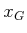and can be interpreted in motion planning applications as the goal state. Stability characterizes howis approached from other states inby integrating the vector field.

The given vector field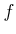is considered as a velocity field, which is represented as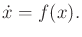(15.1)

This looks like a state transition equation that is missing actions. If a system of the form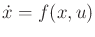is given, thencan be fixed by designing a feedback plan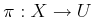. This yields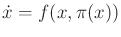, which is a vector field onwithout any further dependency on actions. The dynamic programming approach in Section 14.5 computed such a solution. The process of designing a stable feedback plan is referred to in control literature as feedback stabilization.

Subsections
Steven M LaValle 2020-08-14